# RD Sharma Solutions for Class 11 Chapter 23 - The Straight Lines Exercise 23.17

This exercise discusses the concept based on the area of parallelogram. Students who wish to develop a strong command over the concepts of Maths can make use of RD Sharma Solutions. Students can refer to RD Sharma Class 11 Maths Solutions, which is one of the best reference guides any student can rely on. This resource can also be used by students for preparation of their exams. All the solutions are prepared by subject experts at BYJU’S to match all levels of students. RD Sharma Solutions are made readily available in the pdf format, where any student can download easily, from the links provided below.

## Download the pdf of RD Sharma Solutions for Class 11 Maths Exercise 23.17 Chapter 23 – The Straight Lines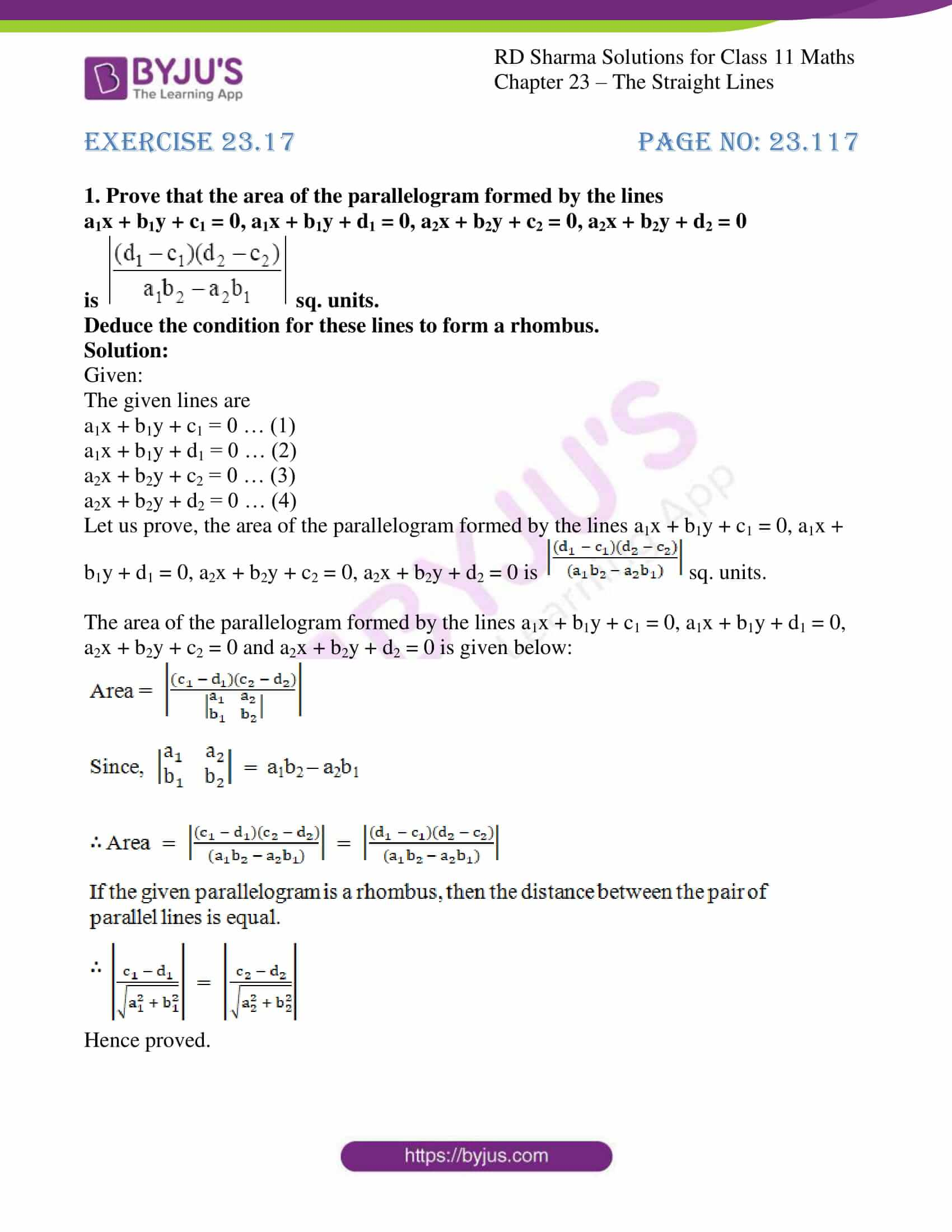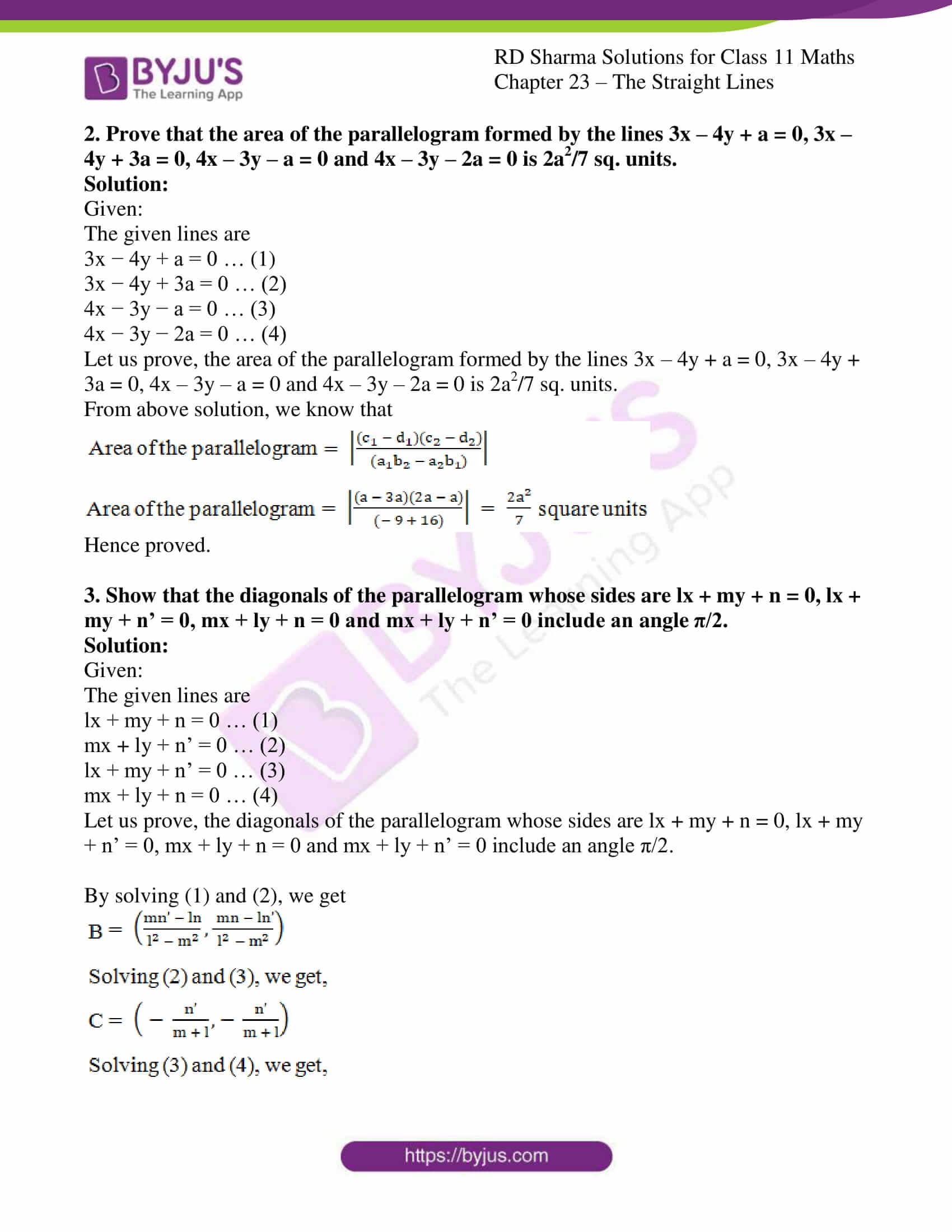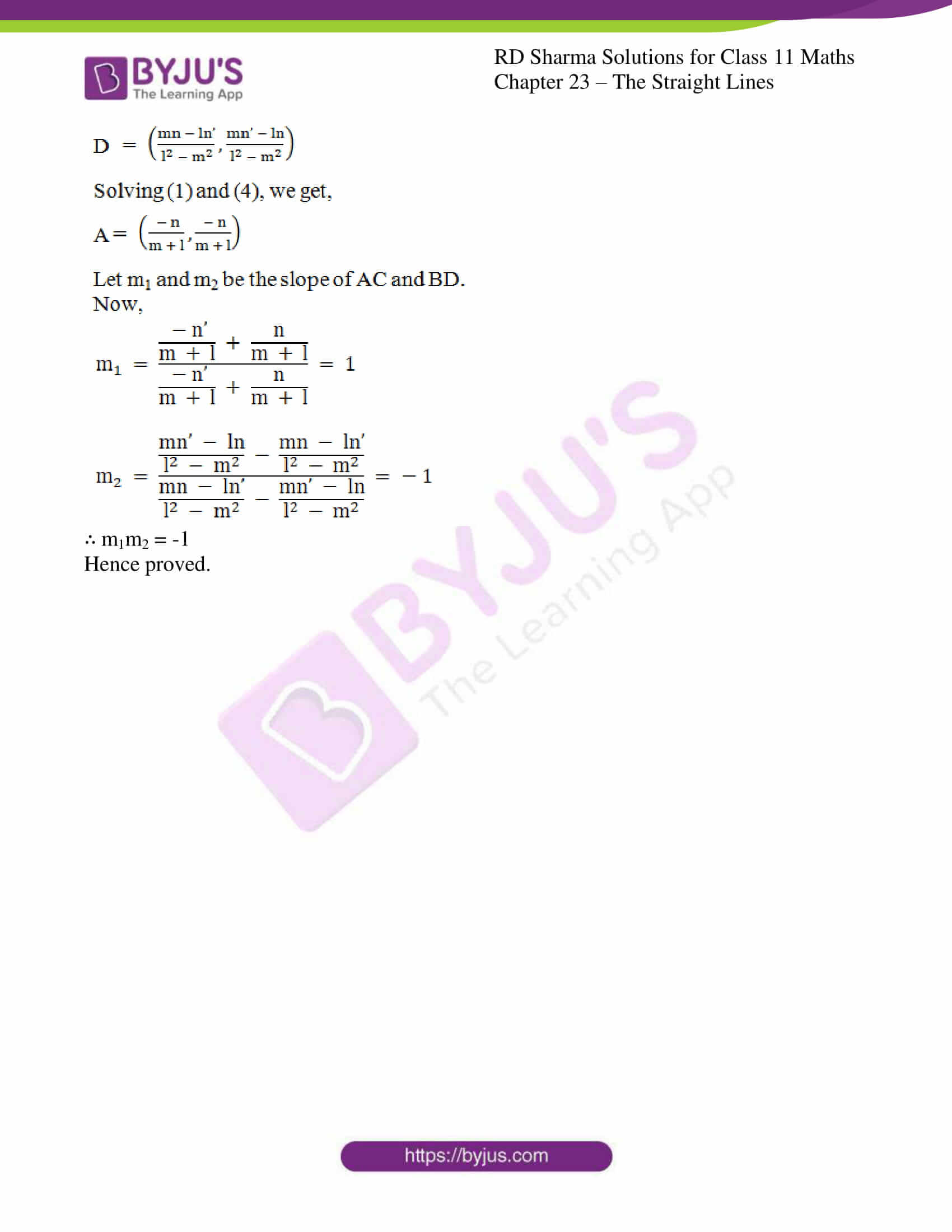### Access answers to RD Sharma Solutions for Class 11 Maths Exercise 23.17 Chapter 23 – The Straight Lines

#### EXERCISE 23.17 PAGE NO: 23.117

1. Prove that the area of the parallelogram formed by the lines
a1x + b1y + c1 = 0, a1x + b1y + d1 = 0, a2x + b2y + c2 = 0, a2x + b2y + d2 = 0 is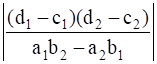sq. units.
Deduce the condition for these lines to form a rhombus.

Solution:

Given:

The given lines are

a1x + b1y + c1 = 0 … (1)

a1x + b1y + d1 = 0 … (2)

a2x + b2y + c2 = 0 … (3)

a2x + b2y + d2 = 0 … (4)

Let us prove, the area of the parallelogram formed by the lines a1x + b1y + c1 = 0, a1x + b1y + d1 = 0, a2x + b2y + c2 = 0, a2x + b2y + d2 = 0 is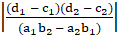sq. units.

The area of the parallelogram formed by the lines a1x + b1y + c1 = 0, a1x + b1y + d1 = 0, a2x + b2y + c2 = 0 and a2x + b2y + d2 = 0 is given below: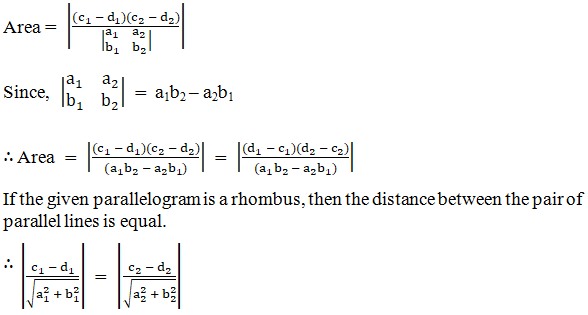Hence proved.

2. Prove that the area of the parallelogram formed by the lines 3x – 4y + a = 0, 3x –4y + 3a = 0, 4x – 3y – a = 0 and 4x – 3y – 2a = 0 is 2a2/7 sq. units.

Solution:

Given:

The given lines are

3x − 4y + a = 0 … (1)

3x − 4y + 3a = 0 … (2)

4x − 3y − a = 0 … (3)

4x − 3y − 2a = 0 … (4)

Let us prove, the area of the parallelogram formed by the lines 3x – 4y + a = 0, 3x – 4y + 3a = 0, 4x – 3y – a = 0 and 4x – 3y – 2a = 0 is 2a2/7 sq. units.

From above solution, we know that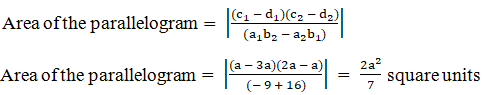Hence proved.

3. Show that the diagonals of the parallelogram whose sides are lx + my + n = 0, lx + my + n’ = 0, mx + ly + n = 0 and mx + ly + n’ = 0 include an angle π/2.

Solution:

Given:

The given lines are

lx + my + n = 0 … (1)

mx + ly + n’ = 0 … (2)

lx + my + n’ = 0 … (3)

mx + ly + n = 0 … (4)

Let us prove, the diagonals of the parallelogram whose sides are lx + my + n = 0, lx + my + n’ = 0, mx + ly + n = 0 and mx + ly + n’ = 0 include an angle π/2.

By solving (1) and (2), we get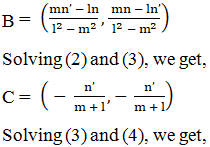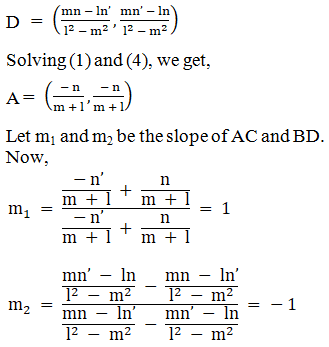∴ m1m2 = -1

Hence proved.